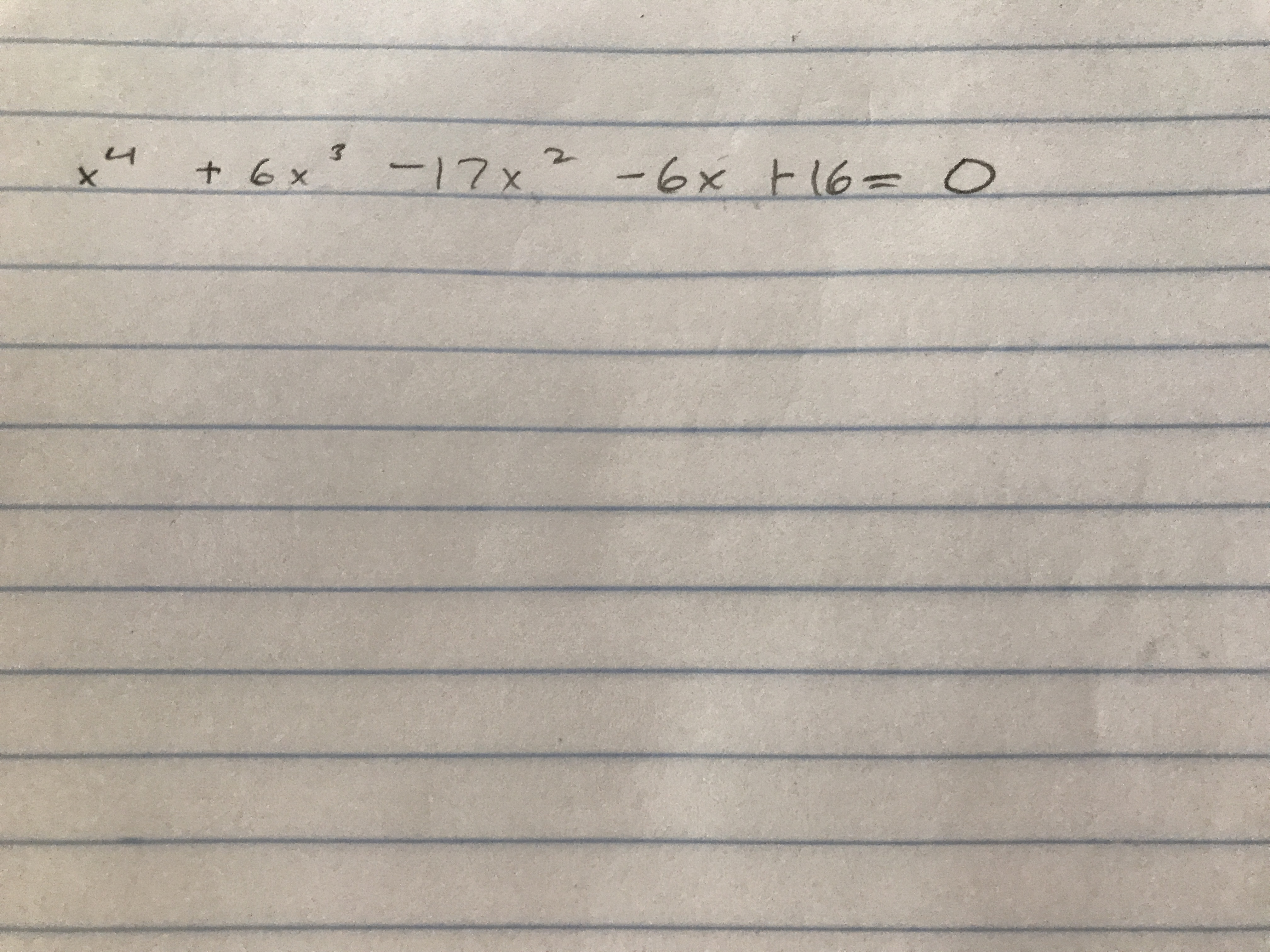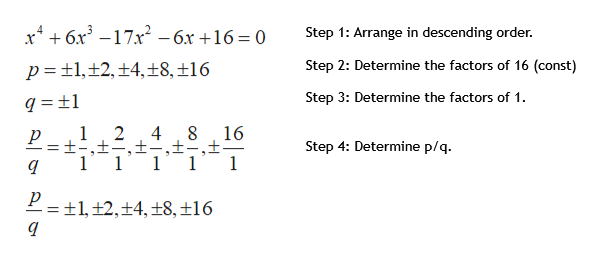# 3+ 6x17 x6x t (6 O

Question

how do i use the rational zero theorem to find all real zeros ? step by step pleasehelp_outlineImage Transcriptionclose3 + 6x 17 x 6x t (6 O fullscreen
check_circleExpert Solution
Step 1

By using Rational Zero theorem to find the all real zeroes of the given polynomial equation.

Step 2

According to "Rational Zeros Theorem":

If f(x) is a polynomial with integer coefficients and if p/q is a zero of f(x) (f(p/q) = 0), then p is a factor of the constant term of f(x) and q is a factor of the leading coefficient of f(x).

We can use the Rational Zeros Theorem to find all the rational zeros of a polynomial. Here are the steps:

Step 1: Write the given polynomial in descending order.

Step 2: Find the factors of constant term named as p.

Step 3: Find the factors of leading coefficient named as q.

Step 4: Write down the p/q (both negative & positive) and cross out the duplicates values.

Step 5: Determine which possible zeros are actual zeros by evaluating each case of f(p/q)

Step 3

Use the steps to determine ...help_outlineImage Transcriptionclosex46x3 -17x Step 1: Arrange in descending order -6x +16 = 0 Step 2: Determine the factors of 16 (const) t1,12, +4,±8, ±16 p Step 3: Determine the factors of 1 1 2 4 16 ±-,± 1 Step 4: Determine p/q 1 1 1 P= 1, ±2,+4,±8,±16 fullscreen

### Want to see the full answer?

See Solution

#### Want to see this answer and more?

Solutions are written by subject experts who are available 24/7. Questions are typically answered within 1 hour*

See Solution
*Response times may vary by subject and question
Tagged in

### Functions